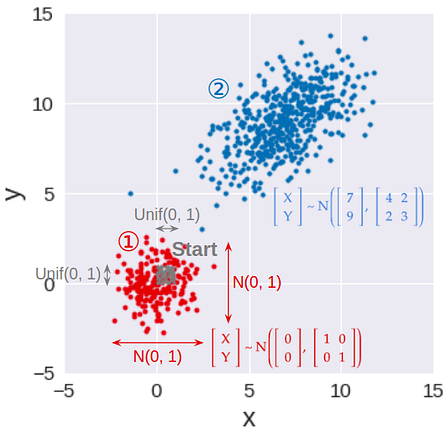# Gaussian samples – Part(1)

## Background

Gaussian sampling — that is, generating samples from a Gaussian distribution — plays an important role in many cutting-edge fields of data science, such as Gaussian processvariational autoencoder, or generative adversarial network. As a result, you often see functions like tf.random.normal in their tutorials.

But, deep down, how does a computer know how to generate Gaussian samples? This series of blog posts will show 3 different ways that we can program our computer (via Python) to do so. You will also see how R and Python generate Gaussian samples using modified versions of some of these methods.

## Starting point: the uniform number generator

Of course, we can’t generate Gaussian samples from thin air. Instead, we start with a random number generator that exists in almost all programming languages: the uniform random number generator. It generates a random number that could take any value between 0 and 1. For Python, the numpy.random module uses the Mersenne twister to generate a uniformly-distributed float that is in the interval [0.0, 1.0).

Since Gaussians are better visualized in 2 dimensions — we are all familiar with the Gaussian “blob” in the xy-plane — I will demonstrate the 3 sampling methods in 2-D, especially since one of the methods do in fact generate Gaussians in two dimensions at the same time.Step 1: Generate standard Gaussian samples in 2-D. Step 2: Transform standard Gaussian samples to have given means, variances, and covariance between x and y

As a result, this series is broken down into 3 parts (see accompanying image):

## Method 1: Inverse transform sampling

This is the most basic, yet most common, way to convert a uniform random sample into a random sample of any distribution, including Gaussian. This method works by applying the inverse function of the Gaussian CDF (cumulative distribution function) to transform a uniform sample to a Gaussian sample.

To make sure that the Gaussian samples for the x- and y-coordinates are independent, we can use two different uniform samples, one for x (U₁), and one for y (U₂). These two uniform samples can be generated using two different random number generators (two different `RandomState` initialized by different seeds, for example) so that they are independent in the first place.